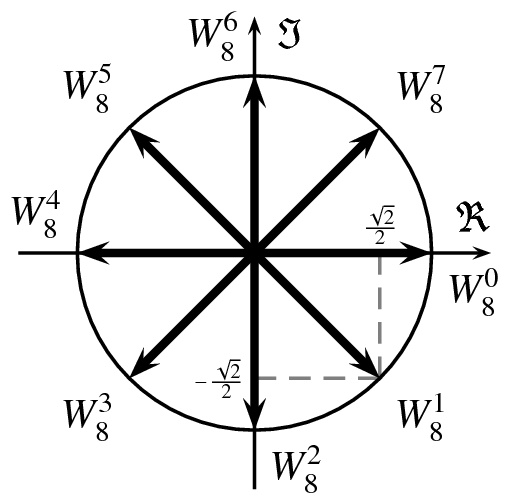# A Complex problem

Algebra Level 3Let $\{\phi_i\}_1^{n-1}$ denote the set of solutions to the equation : $\sum_{k=0}^{n-1} \ z^k = 0, \quad where \ n >1 \ and \ \ z \in \mathbb C$

What is the value of $\displaystyle \sum_{k=1}^{n-1} \ \phi_k$?

×

Problem Loading...

Note Loading...

Set Loading...